# KVPY-SA 2017 Chemistry Paper with Solutions

KVPY-SA 2017 Chemistry paper with solutions is a perfect resource which helps the candidates in understanding the different approaches that are used in solving a certain problem. Candidates can download the previous years’ question papers of KVPY-SA to figure out the nature of questions that can appear in the main examination. Practising the KVPY-SA previous years’ papers will assist the students in obtaining in-depth knowledge about the topics and also introduce them to the important ones. Solving these papers with solutions will help the students to understand the pattern of the Chemistry question paper for the KVPY exam.

### KVPY SA 2017 - Chemistry

Question 1: The structure of 3-methylpent-2-ene is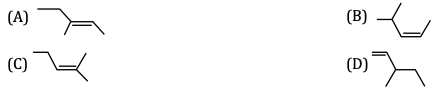Solution: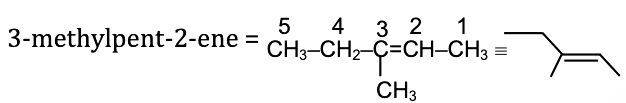Question 2: The stability of carbanions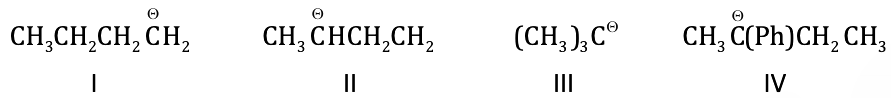follows the order

1. a. III < IV < I < II
2. b. I < II < IV < III
3. c. III < II < I < IV
4. d. IV < III < II < I

Solution: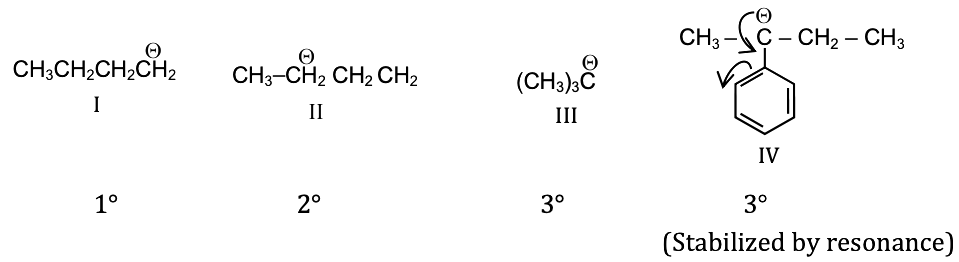And more the +I effect/hyperconjugation effect less will be the stability of carbanion.

Therefore 1° > 2° > 3° (stability of carbanion)

Hence overall order IV > I > II > III.

Question 3: In the following reaction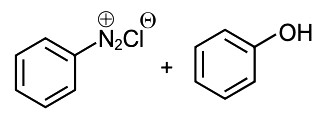The major product is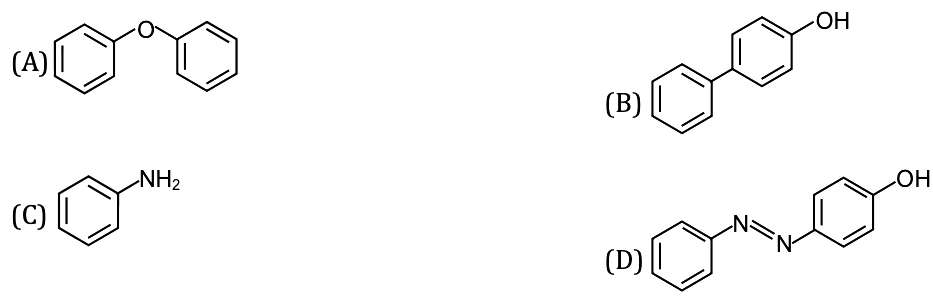Solution: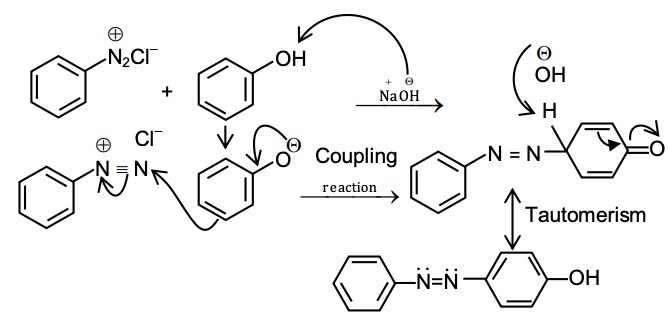Question 4: In the reaction of 1-bromo-3-Chlorocyclobutane with two equivalents of sodium in ether, the major product is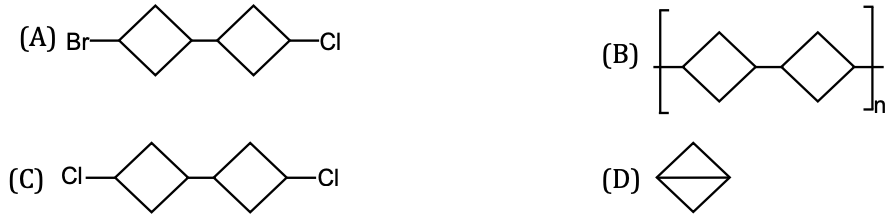Solution: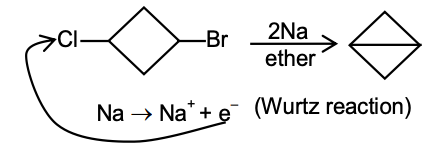Question 5: The order of basicity of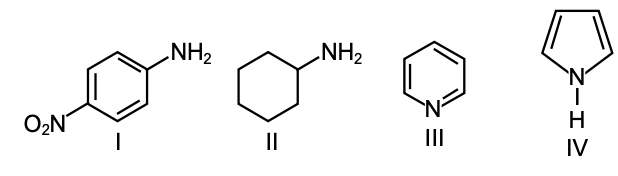in water is

1. a. IV < III < I < II
2. b. II < I < IV < III
3. c. IV < I < III < II
4. d. II < III < I < IV

Solution:

More the tendency of donating lone pair more will be the basicity.

More the lone pair involved in resonance less will be the basicity.

More electronegativity, less basicity.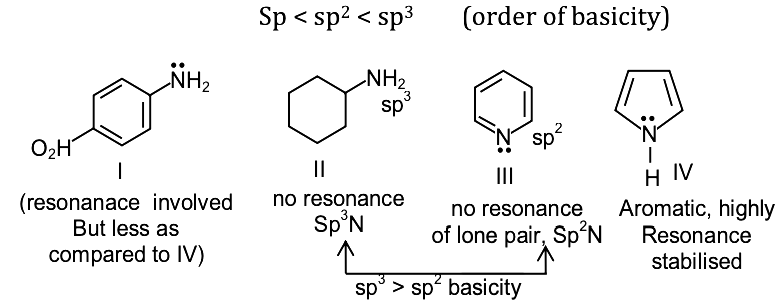Hence overall order of basicity II > III > I > IV.

Question 6: The first ionisation energy of Na, B, N and O atoms follows the order

1. a. B < Na < O < N
2. b. Na < B < O < N
3. c. Na < O < B < N
4. d. O < Na < N < B

Solution:

Na →1s2 2s2 2p6 3s1→ will attain noble gas configuration after removing one e → least I.E.

B → 1s2 2s2 2p1

N → 1s2 2s2 2p3 → half- filled stabilized → highest I.E.

O → 1s2 2s2 2p4

In between B and O

Along the period size ↓ I.E. ↑.

Na < B < O < N

Question 7: Among P2O5, As2O3, Sb2O3 and Bi2O3 the most acidic oxide is

1. a. P2O5
2. b. As2O3
3. c. Sb2O3
4. d. Bi2O3

Solution:

+5P2O5

+3As2O3

+3Sb2O3

+3Bi2O3

More the oxidation number more will be the tendency to accept lone pair of e → more will be the acidic character.

OR

Down the group, metallic character increase, hence the basic character of oxides increases. Hence P2O5 is the most acidic oxide.

Question 8: Among K, Mg, Au and Cu, the one which is extracted by heating its ore in the air is

1. a. K
2. b. Mg
3. c. Au
4. d. Cu

Solution:

2CuFeS2 + 2SiO2 + 4O2 → Cu2S + 2FeSiO3 + 3SO2

Cu2S + O2 → 2Cu + SO2

Question 9: The metal ion with the total number of electrons same as S2– is

1. a. Na+
2. b. Ca2+
3. c. Mg2+
4. d. Sr2+

Solution:

Given:-

S2– → 18 e

From the options,

Na+ → 10e

Ca2+ → 18e

Mg2+ → 10e

Sr2+ → 36 e

Ca2+ and S2– have the same number of electrons.

Question 10: X g of Ca [atomic mass = 40] dissolves completely in concentrated HCl solution to produce 5.04 L of H2 gas at STP. The value of X is closest to

1. a. 4.5
2. b. 8.1
3. c. 9.0
4. d. 16.2

Solution:

1. Answer: (C)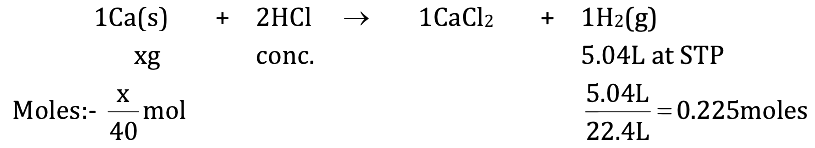Apply mole – mole analysis:-

1 mole of Ca produce → 1 mole of H2(g)

[x / 40] moles of Ca will produce → [x / 40] moles of H2(g)

Given: [x / 40] = 0.225

= 9.0

Question 11: A 20 g object is moving with a velocity of 100 ms–1. The de Broglie wavelength (in m) of the object is [Planck’s constant h = 6.626 × 10–34 J s]

1. a. 3.313 × 10–34
2. b. 6.626 × 10–34
3. c. 3.313 × 10–31
4. d. 6.626 × 10–31

Solution:

Given, m = 20g, v = 100 ms–1

De Broglie wavelength → λ = h / mv = [6.626 × 10–34 J s] / [20 * 10-3 kg * 100ms-1]

Units:

m → kg

v → m / s

h → Js

then λ → (m)

λ = 3.313 × 10–34 m

Question 12: In a closed vessel at STP, 50 L of CH4 is ignited with 750 L of air (containing 20% O2). The number of moles of O2 remaining in the vessel on cooling to room temperature is closest to

1. a. 5.8
2. b. 2.2
3. c. 4.5
4. d. 6.7

Solution:

1CH4 + 2O2 → CO2 + 2H2O

Air → 750L

20% O2 → [20 * 750] /  = 150L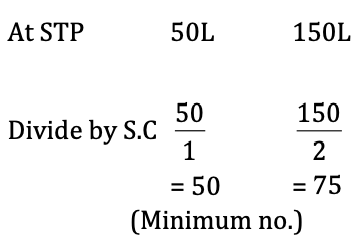Hence, CH4 is the limiting reagent (consumed completely).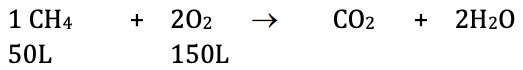After the reaction

0 150 – 100 50L 100L

= 50L

Volume of O2 remained = 50L

Moles of O2 remained = 50L / 22.4L = 2.2moles

Question 13: CO2 is passed through lime water. Initially, the solution turns milky and then becomes clear upon continued bubbling of CO2. The clear solution is due to the formation of

1. a. CaCO3
2. b. CaO
3. c. Ca(OH)2
4. d. Ca(HCO3)2

Solution:

Ca(OH)2 + CO2 → CaCO3 + H2O

(turn milky) → because of the formation of CaCO3 ppt

CaCO3 + H2 O $\overset{CO_2}{\rightarrow}$ Ca(HCO3)2

Clear solution because of the formation of soluble Ca(HCO3)2

Question 14: The maximum number of electrons that can be filled in the shell with the principal quantum number n = 3 is

1. a. 18
2. b. 9
3. c. 8
4. d. 2

Solution:

n = 3

Then possible subshell =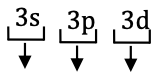Maximum electrons occupied → 2 + 6 + 10 = 18 electrons

Question 15: The atomic radii of Li, F, Na and Si follow the order

1. a. Si > Li > Na > F
2. b. Li > F > Si > Na
3. c. Na > Si > F > Li
4. d. Na > Li > Si > F

Solution: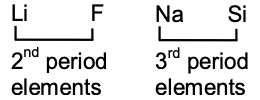→ Size of 3rd period element > size of 2nd element period

→ along the period → size ↓

Overall order

Na > Li > Si > F

Question 16: The reaction of an alkene X with bromine produces a compound Y, which has 22.22% C, 3.71% H and 74.07% Br. The ozonolysis of alkene X gives only one product. The alkene X is: [Given: atomic mass of C = 12; H = 1; Br = 80]

1. a. Ethylene
2. b. 1-butene
3. c. 2-butene
4. d. 3-hexene

Solution:

 % Atomic man Relative no of atoms (Whole no) ratio of atoms C 22.22 12 22.22 / 12 = 1.85 1.85 / 0.925 = 2 H 3.71 1 3.71 / 1 = 3.71 3.71 / 0.925 = 4 Br 74.07 SO 74.07 / 80 = 0.925 0.925 / 0.925 = 1

Hence empirical formula of Y = C2H4Br

On Ozonolysis of X → it gives only one product.

Hence the correct option is C (2 – butene).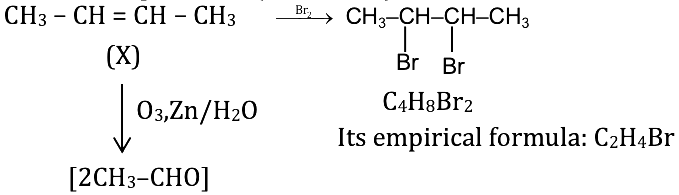Question 17: In the following reaction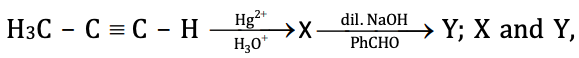respectively, are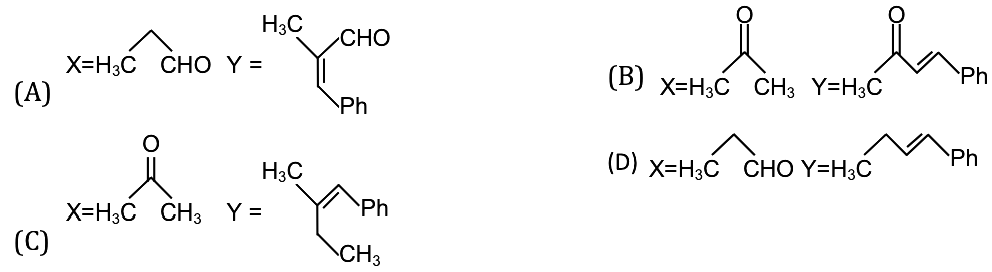Solution: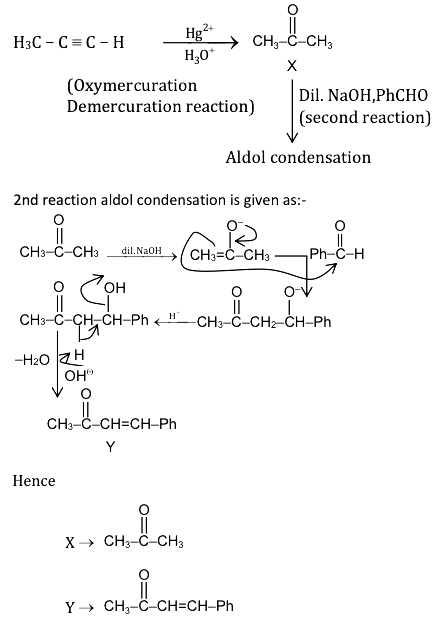Question 18: KMnO4 reacts with H2O2 in an acidic medium. The number of moles of oxygen produced per mole of KMnO4 is

1. a. 2.5
2. b. 5
3. c. 1.25
4. d. 2

Solution: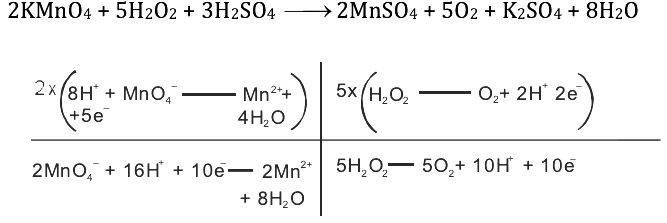2MnO4- + 5H2O2 + 8H+ → 2Mn2+ + 5O2 + 8H2O

From the reaction

2 mole of KMnO4 produce → 5 moles of O2

1 mole of KMnO4 produce → 2.5 moles of O2

Hence mole of O2 produced / mole of KMnO4 = 2.5

Question 19: The photoelectric behaviour of K, Li, Mg and Ag metals is shown in the plot below. If the light of wavelength 400 nm is incident on each of these metals, which of them will emit photoelectrons?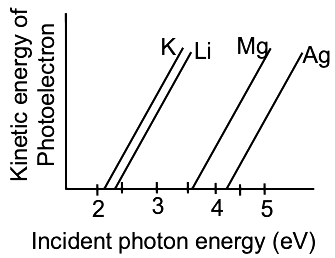1. a. K
2. b. K and Li
3. c. K, Li and Mg
4. d. K, Li, Mg and Ag

Solution: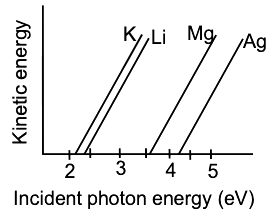Incident light λ = 400nm.

Energy of incident light = 12400 eV / 4000Ao = 3.1 eV

If the energy of incident light ≥ work function then photoelectron will be ejected. From the graph,

In the case of K, Li→ work function < 3.1 eV: hence photoelectrons will be emitted.

But in case of Mg, Ag → work function > 3.1 eV: hence no photoelectrons will be emitted.

Question 20: A piece of metal weighing 100 g is heated to 80°C and dropped into 1 kg of cold water in an insulated container at 15°C. If the final temperature of the water in the container is 15.69°C. If the final temperature of the water in the container is 15.69°C, the specific heat of the metal in J/g °C is

1. a. 0.38
2. b. 0.24
3. c. 0.45
4. d. 0.13

Solution:

For metal,

M = 100 g

T1 = 80°C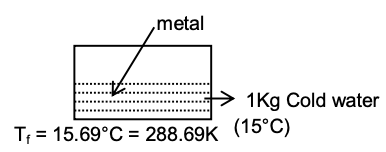Heat loss by metal = Heat gain by cold water

– m1S1 (Tf – T1) = m2S2 (Tf – T2)

– 100S1 (288.69 – 353) = 1000 × 4.2 (288.69 – 288)

S1 = 0.45 J/g°C

(Specific heat of metal)

### KVPY-SA 2017 Chemistry Paper with Solutions